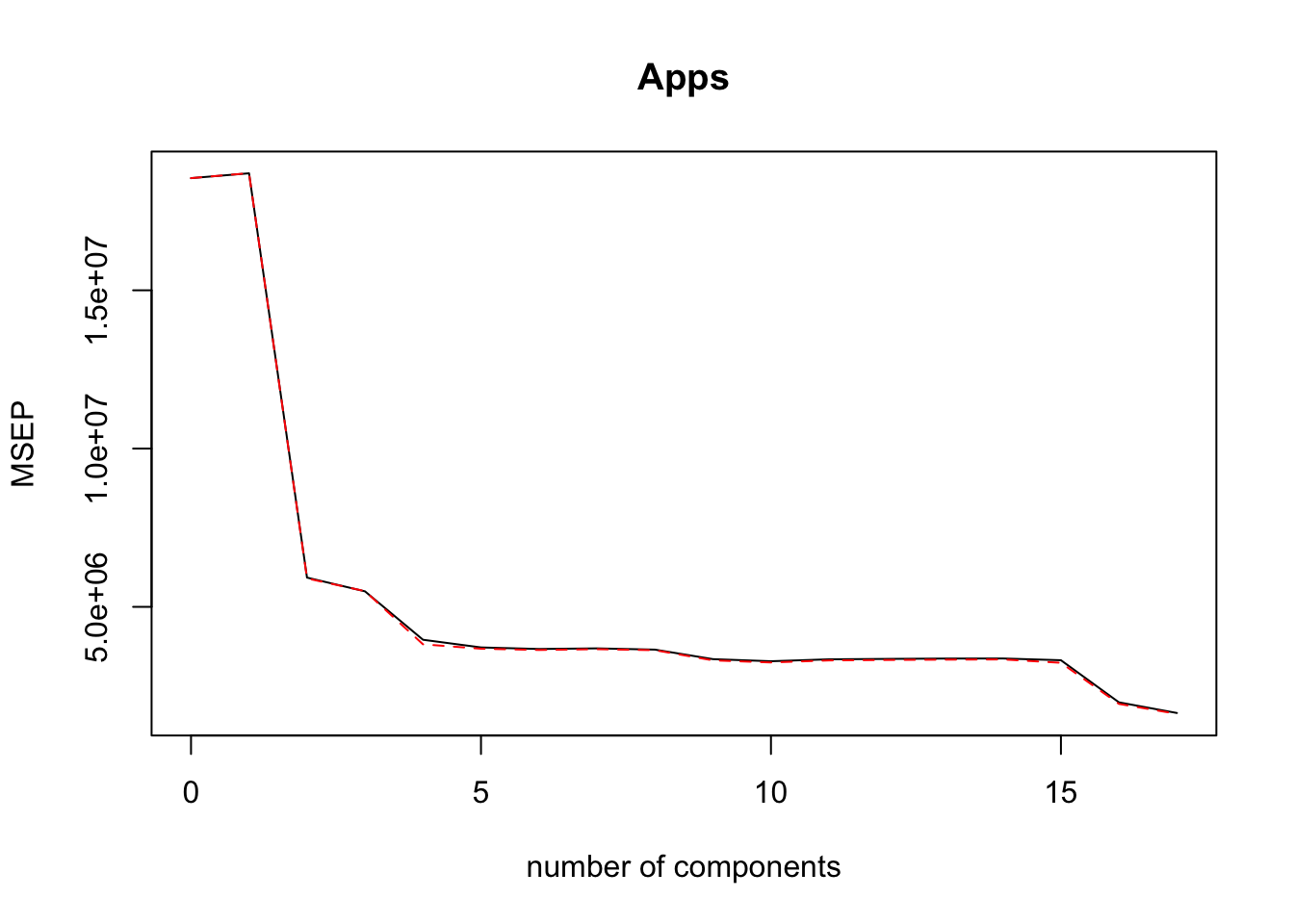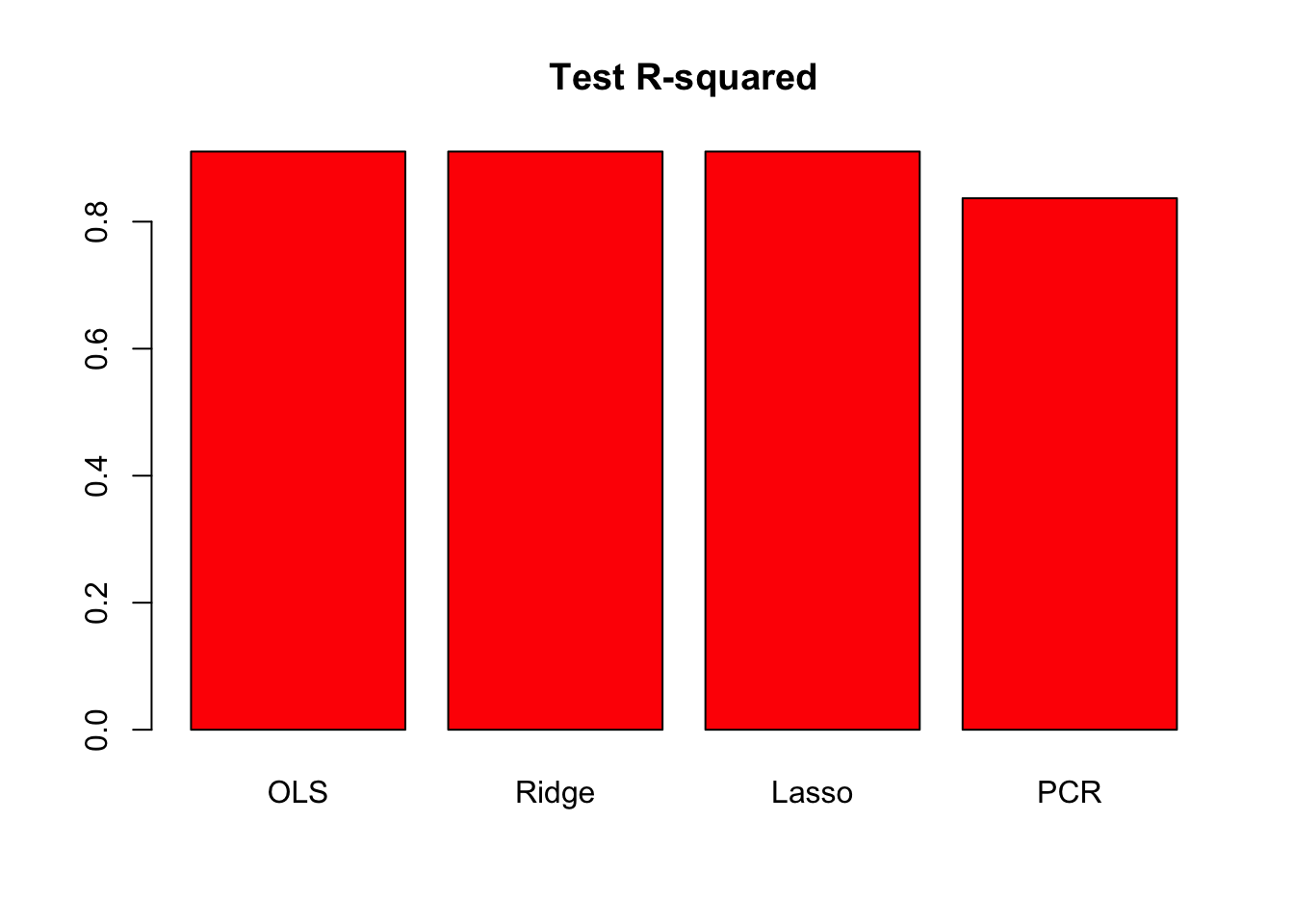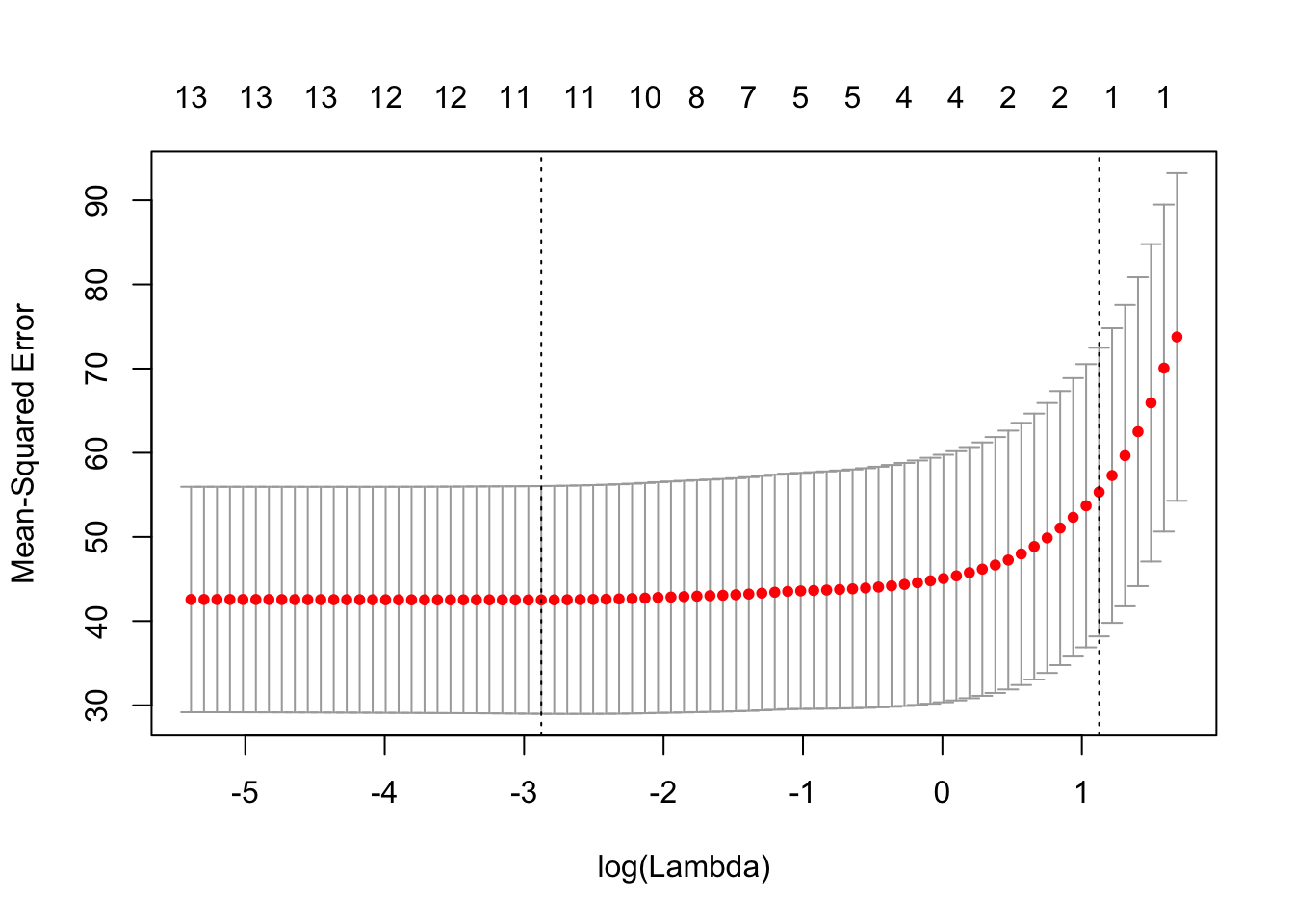You will need to load the core library for the course textbook:

library(ISLR)

## Exercise 6.1

In the lab session for this topic (Sections 5.3.2 and 5.3.3 in James et al.), we saw that the cv.glm() function can be used in order to compute the LOOCV test error estimate. Alternatively, one could compute those quantities using just the glm() and predict.glm() functions, and a for loop. You will now take this approach in order to compute the LOOCV error for a simple logistic regression model on the Weekly data set. Recall that in the context of classification problems, the LOOCV error is given in Section 5.1.5 (5.4, page 184).

library(ISLR)
summary(Weekly)
##       Year           Lag1               Lag2               Lag3
##  Min.   :1990   Min.   :-18.1950   Min.   :-18.1950   Min.   :-18.1950
##  1st Qu.:1995   1st Qu.: -1.1540   1st Qu.: -1.1540   1st Qu.: -1.1580
##  Median :2000   Median :  0.2410   Median :  0.2410   Median :  0.2410
##  Mean   :2000   Mean   :  0.1506   Mean   :  0.1511   Mean   :  0.1472
##  3rd Qu.:2005   3rd Qu.:  1.4050   3rd Qu.:  1.4090   3rd Qu.:  1.4090
##  Max.   :2010   Max.   : 12.0260   Max.   : 12.0260   Max.   : 12.0260
##       Lag4               Lag5              Volume
##  Min.   :-18.1950   Min.   :-18.1950   Min.   :0.08747
##  1st Qu.: -1.1580   1st Qu.: -1.1660   1st Qu.:0.33202
##  Median :  0.2380   Median :  0.2340   Median :1.00268
##  Mean   :  0.1458   Mean   :  0.1399   Mean   :1.57462
##  3rd Qu.:  1.4090   3rd Qu.:  1.4050   3rd Qu.:2.05373
##  Max.   : 12.0260   Max.   : 12.0260   Max.   :9.32821
##      Today          Direction
##  Min.   :-18.1950   Down:484
##  1st Qu.: -1.1540   Up  :605
##  Median :  0.2410
##  Mean   :  0.1499
##  3rd Qu.:  1.4050
##  Max.   : 12.0260
set.seed(1)
1. Fit a logistic regression model that predicts Direction using Lag1 and Lag2.
glm.fit <-  glm(Direction ~ Lag1 + Lag2,
data = Weekly,
family = binomial)
summary(glm.fit)
##
## Call:
## glm(formula = Direction ~ Lag1 + Lag2, family = binomial, data = Weekly)
##
## Deviance Residuals:
##    Min      1Q  Median      3Q     Max
## -1.623  -1.261   1.001   1.083   1.506
##
## Coefficients:
##             Estimate Std. Error z value Pr(>|z|)
## (Intercept)  0.22122    0.06147   3.599 0.000319 ***
## Lag1        -0.03872    0.02622  -1.477 0.139672
## Lag2         0.06025    0.02655   2.270 0.023232 *
## ---
## Signif. codes:  0 '***' 0.001 '**' 0.01 '*' 0.05 '.' 0.1 ' ' 1
##
## (Dispersion parameter for binomial family taken to be 1)
##
##     Null deviance: 1496.2  on 1088  degrees of freedom
## Residual deviance: 1488.2  on 1086  degrees of freedom
## AIC: 1494.2
##
## Number of Fisher Scoring iterations: 4
1. Fit a logistic regression model that predicts Direction using Lag1 and Lag2 using all but the first observation.
glm.fit <-  glm(Direction ~ Lag1 + Lag2,
data = Weekly[-1,],
family = binomial)

summary(glm.fit)
##
## Call:
## glm(formula = Direction ~ Lag1 + Lag2, family = binomial, data = Weekly[-1,
##     ])
##
## Deviance Residuals:
##     Min       1Q   Median       3Q      Max
## -1.6258  -1.2617   0.9999   1.0819   1.5071
##
## Coefficients:
##             Estimate Std. Error z value Pr(>|z|)
## (Intercept)  0.22324    0.06150   3.630 0.000283 ***
## Lag1        -0.03843    0.02622  -1.466 0.142683
## Lag2         0.06085    0.02656   2.291 0.021971 *
## ---
## Signif. codes:  0 '***' 0.001 '**' 0.01 '*' 0.05 '.' 0.1 ' ' 1
##
## (Dispersion parameter for binomial family taken to be 1)
##
##     Null deviance: 1494.6  on 1087  degrees of freedom
## Residual deviance: 1486.5  on 1085  degrees of freedom
## AIC: 1492.5
##
## Number of Fisher Scoring iterations: 4
1. Use the model from (b) to predict the direction of the first observation. You can do this by predicting that the first observation will go up if P(Direction="Up"|Lag1, Lag2) > 0.5. Was this observation correctly classified?
predict.glm(glm.fit, Weekly[1,], type="response") > 0.5
##    1
## TRUE

Prediction was UP, true Direction was DOWN.

1. Write a for loop from i=1 to i=n, where n is the number of observations in the data set, that performs each of the following steps:
i. Fit a logistic regression model using all but the i-th observation to predict Direction using Lag1 and Lag2.

ii. Compute the posterior probability of the market moving up for the i-th observation.

iii. Use the posterior probability for the i-th observation in order to predict whether or not the market moves up.

iv. Determine whether or not an error was made in predicting the direction for the i-th observation. If an error was made, then indicate this as a 1, and otherwise indicate it as a 0.
count <-rep(0, nrow(Weekly))

for (i in 1:nrow(Weekly)) {
glm.fit <-  glm(Direction ~ Lag1 + Lag2,
data = Weekly[-i,],
family = binomial)

is_up <-  predict.glm(glm.fit, Weekly[i,], type="response") > 0.5
is_true_up <-  Weekly[i,]$Direction == "Up" if (is_up != is_true_up) count[i] <- 1 } sum(count) ##  490 490 errors. 1. Take the average of the n numbers obtained in (d)iv in order to obtain the LOOCV estimate for the test error. Comment on the results. mean(count) ##  0.4499541 LOOCV estimates a test error rate of 45%. ## Exercise 6.2 In this exercise, we will predict the number of applications received using the other variables in the College data set. 1. Split the data set into a training set and a test set. Load and split the College data. library(ISLR) set.seed(11) sum(is.na(College)) ##  0 train.size <- nrow(College) / 2 train <- sample(1:nrow(College), train.size) test <- -train College.train <- College[train, ] College.test <- College[test, ] 1. Fit a linear model using least squares on the training set, and report the test error obtained. Number of applications is the Apps variable. lm.fit <- lm(Apps ~ . , data = College.train) lm.pred <- predict(lm.fit, College.test) mean((College.test[, "Apps"] - lm.pred)^2) ##  1026096 Test RSS is 1538442 1. Fit a ridge regression model on the training set, with $$\lambda$$ chosen by cross-validation. Report the test error obtained. Pick $$\lambda$$ using College.train and report error on College.test library(glmnet) ## Loading required package: Matrix ## Loading required package: foreach ## Loaded glmnet 2.0-18 train.mat <- model.matrix(Apps ~ . , data = College.train) test.mat <- model.matrix(Apps ~ . , data = College.test) grid <- 10 ^ seq(4, -2, length = 100) mod.ridge <- cv.glmnet(train.mat, College.train[, "Apps"], alpha = 0, lambda = grid, thresh = 1e-12) lambda.best <- mod.ridge$lambda.min
lambda.best
##  0.01
ridge.pred <-  predict(mod.ridge, newx = test.mat, s = lambda.best)
mean((College.test[, "Apps"] - ridge.pred)^2)
##  1026069

Test RSS is slightly higher than OLS, 1608859.

1. Fit a lasso model on the training set, with $$\lambda$$ chosen by cross-validation. Report the test error obtained, along with the number of non-zero coefficient estimates.

Pick $$\lambda$$ using College.train and report error on College.test.

mod.lasso <-  cv.glmnet(train.mat, College.train[, "Apps"],
alpha = 1, lambda = grid, thresh = 1e-12)
lambda.best <-  mod.lasso$lambda.min lambda.best ##  0.01 lasso.pred <- predict(mod.lasso, newx = test.mat, s = lambda.best) mean((College.test[, "Apps"] - lasso.pred)^2) ##  1026036 Again, Test RSS is slightly higher than OLS, 1635280. The coefficients look like mod.lasso <- glmnet(model.matrix(Apps ~ . , data = College), College[, "Apps"], alpha = 1) predict(mod.lasso, s = lambda.best, type = "coefficients") ## 19 x 1 sparse Matrix of class "dgCMatrix" ## 1 ## (Intercept) -471.39372069 ## (Intercept) . ## PrivateYes -491.04485135 ## Accept 1.57033288 ## Enroll -0.75961467 ## Top10perc 48.14698891 ## Top25perc -12.84690694 ## F.Undergrad 0.04149116 ## P.Undergrad 0.04438973 ## Outstate -0.08328388 ## Room.Board 0.14943472 ## Books 0.01532293 ## Personal 0.02909954 ## PhD -8.39597537 ## Terminal -3.26800340 ## S.F.Ratio 14.59298267 ## perc.alumni -0.04404771 ## Expend 0.07712632 ## Grad.Rate 8.28950241 1. Fit a PCR model on the training set, with $$M$$ chosen by cross-validation. Report the test error obtained, along with the value of $$M$$ selected by cross-validation. Use validation to fit PCR library(pls) ## ## Attaching package: 'pls' ## The following object is masked from 'package:stats': ## ## loadings pcr.fit <- pcr(Apps ~ . , data = College.train, scale = TRUE, validation = "CV") validationplot(pcr.fit, val.type="MSEP")pcr.pred <- predict(pcr.fit, College.test, ncomp=10) mean((College.test[, "Apps"]- pcr.pred)^2) ##  1867486 Test RSS for PCR is about 3014496. 1. Comment on the results obtained. How accurately can we predict the number of college applications received? Is there much difference among the test errors resulting from these five approaches? Results for OLS, Lasso, Ridge are comparable. Lasso reduces the $$F.Undergrad$$ and $$Books$$ variables to zero and shrinks coefficients of other variables. Here are the test $$R^2$$ for all models. test.avg <- mean(College.test[, "Apps"]) lm.test.r2 <- 1 - mean((College.test[, "Apps"] - lm.pred)^2) / mean((College.test[, "Apps"] - test.avg)^2) ridge.test.r2 <- 1 - mean((College.test[, "Apps"] - ridge.pred)^2)/ mean((College.test[, "Apps"] - test.avg)^2) lasso.test.r2 <- 1 - mean((College.test[, "Apps"] - lasso.pred)^2) / mean((College.test[, "Apps"] - test.avg)^2) pcr.test.r2 <- 1 - mean((College.test[, "Apps"] - pcr.pred)^2) / mean((College.test[, "Apps"] - test.avg)^2) barplot(c(lm.test.r2, ridge.test.r2, lasso.test.r2, pcr.test.r2), col = "red", names.arg = c("OLS", "Ridge", "Lasso", "PCR"), main = "Test R-squared")The plot shows that test $$R^2$$ for all models except PCR are around 0.9. PCR has a smaller test $$R^2$$ of less than 0.8. All models except PCR predict college applications with high accuracy. ## Exercise 6.3 (Optional) We will now try to predict per capita crime rate in the Boston data set. 1. Try out some of the regression methods explored in this chapter, such as best subset selection, the lasso, ridge regression, and PCR. Present and discuss results for the approaches that you consider. set.seed(1) library(MASS) library(leaps) library(glmnet) Lasso x <- model.matrix(crim ~ . -1, data = Boston) y <- Boston$crim
cv.lasso <-  cv.glmnet(x, y, type.measure = "mse")
plot(cv.lasso)coef(cv.lasso)
## 14 x 1 sparse Matrix of class "dgCMatrix"
##                     1
## (Intercept) 1.0894283
## zn          .
## indus       .
## chas        .
## nox         .
## rm          .
## age         .
## dis         .
## rad         0.2643196
## tax         .
## ptratio     .
## black       .
## lstat       .
## medv        .
sqrt(cv.lasso$cvm[cv.lasso$lambda == cv.lasso$lambda.1se]) ##  7.438669 Ridge regression x <- model.matrix(crim ~ . -1, data = Boston) y <- Boston$crim
cv.ridge <-  cv.glmnet(x, y, type.measure = "mse", alpha = 0)
plot(cv.ridge)